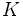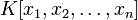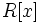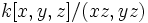Difference between revisions of "Cohen-Macaulay ring"

This article is about a definition in group theory that is standard among the commutative algebra community (or sub-community that dabbles in such things) but is not very basic or common for people outside

View a list of other standard non-basic definitions
This article defines a property of commutative unital rings; a property that can be evaluated for a commutative unital ring
View all properties of commutative unital rings
VIEW RELATED: Commutative unital ring property implications | Commutative unital ring property non-implications |Commutative unital ring metaproperty satisfactions | Commutative unital ring metaproperty dissatisfactions | Commutative unital ring property satisfactions | Commutative unital ring property dissatisfactions

Definition

Symbol-free definition

A commutative unital ring is termed Cohen-Macaulay if it is Noetherian and satisfies the following equivalent conditions:

Equivalence of definitions

Further information: Equivalence of definitions of Cohen-Macaulay

Relation with other properties

Stronger properties

Property Meaning Proof of implication Proof of strictness (reverse implication failure) Intermediate notions
Regular local ring regular local implies Cohen-Macaulay Cohen-Macaulay not implies regular local click here
regular ring its localization at any prime ideal is a regular local ring Regular implies Cohen-Macaulay Cohen-Macaulay not implies regular click here
polynomial ring over a field has the form$K[x]$ where$K$ is a field click here
multivariate polynomial ring over a field has the form$K[x_1,x_2,\dots,x_n]$ where$K$ is a field click here
principal ideal domain every ideal is a principal ideal, i.e., it is generated by one element click here
Artinian ring every descending chain of ideals stabilizes at a finite length Artinian implies Cohen-Macaulay click here
one-dimensional Noetherian domain one-dimensional Noetherian integral domain One-dimensional Noetherian domain implies Cohen-Macaulay click here

Weaker properties

Property Meaning Proof of implication Proof of strictness (reverse implication failure) Intermediate notions
universally catenary ring Cohen-Macaulay implies universally catenary universally catenary not implies Cohen-Macaulay click here

Metaproperties

Closure under taking the polynomial ring

This property of commutative unital rings is polynomial-closed: it is closed under the operation of taking the polynomial ring. In other words, if$R$ is a commutative unital ring satisfying the property, so is$R[x]$

View other polynomial-closed properties of commutative unital rings

A polynomial ring over a Cohen-Macaulay ring is Cohen-Macaulay. For full proof, refer: Cohen-Macaulay is polynomial-closed

Strong local nature

This property of commutative unital rings is strongly local in the following sense: a commutative unital ring has the property iff its localization at each prime ideal has the property, iff its localization at each maximal ideal has the property
View other strongly local properties of commutative unital rings

The property of being a Cohen-Macaulay ring is local in the sense that a commutative unital ring is Cohen-Macaulay if and only if its localizations at all maximal ideals are Cohen-Macaulay, if and only if its localizations at all prime ideals are Cohen-Macaulay. For full proof, refer: Cohen-Macaulay is strongly local

Closure under taking quotients

This property of commutative unital rings is not quotient-closed: in other words, a quotient of a commutative unital ring with this property, need not have this property

A quotient of a Cohen-Macaulay ring by an ideal need not be Cohen-Macaulay. However, if the ideal is generated by a regular sequence, then the quotient is a Cohen-Macaulay ring. Thus, for instance, the quotient by a principal ideal generated by an element which is not a zero divisor, is again a Cohen-Macaulay ring.

Direct products

This property of commutative unital rings is finite direct product-closed: a finite direct product of rings with this property, also has this property
View other finite direct product-closed properties of commutative unital rings

A finite direct product of Cohen-Macaulay rings is Cohen Macaulay. For full proof, refer: Cohen-Macaulay is finite direct product-closed

Spectrum

The spectrum of a Cohen-Macaulay ring has the following important geometric property: If two irreducible components intersect, they must have the same dimension. Here, by the dimension of an irreducible component, we mean the dimension of the corresponding minimal prime ideal, or more explicitly, the Krull dimension of the quotient ring by that minimal prime ideal.

This rules out, for instance, rings like$k[x,y,z]/(xz,yz)$.

However:

• The spectrum need not be irreducible: The ring$k[x,y]/(xy)$ is Cohen-Macaulay, although its spectrum has two irreducible components.
• All components of the spectrum need not have the same dimension: For instance, we could have a ring that is a disjoint union of irreducible subsets of different dimensions
• Not every irreducible space is the spectrum of a Cohen-Macaulay ring. In other words, not every integral domain, or even every affine domain, is Cohen-Macaulay,